2018-01-23 18:42:29 lynn0085 阅读数 1596

#### 泊松分布的特征与应用（概统2.应用）

P {X=k} =$\frac{\left(\lambda {\right)}^{k}}{k!}{e}^{-\lambda }$$\frac{(\lambda )^{k} }{k!} e^{-\lambda }$, k=0,1,2,…### 泊松分布的特征：

1）泊松分布的图形只取决于平均数$\lambda$$\lambda$
2）当$\lambda$$\lambda$很小时，图形是很偏的，但当$\lambda$$\lambda$增大时，图形逐渐趋向正态，当$\lambda$$\lambda$=20时，泊松分布接近正态，当$\lambda$$\lambda$>50时，可以认为是正态分布。
3）由泊松分布的图形示例，可以看得出来，k值在$\lambda$$\lambda$附近时，概率最大，
$k=\lambda$$k=\lambda$，P{X=k}等于峰值
4）泊松分布具有可加性
5）泊松分布的均值和方差相等。

$p\to 0$$p\to0$时，$\left(1-p\right)\to 1$$(1-p)\to1$
$\sigma$$\sigma$ = $\sqrt{np\left(1-p\right)}$$\sqrt{np(1-p)}$ = $\sqrt{np}$$\sqrt{np}$

[实例1] 某厂有300台设备，每台设备发生故障的概率是0.01，每名工人一个时间只能维修一台设备，问需要配备多少名维修工人，才能保证事故发生不能被及时维修的概率小于1%？

n=300,p=0.01, n*p =300*0.01=3; n很大，p很小，n*p=常数，符合泊松分布的要求；
$\lambda =n\ast p=300\ast 0.01=3;$$\lambda = n*p = 300*0.01 =3 ;$k取0~${k}_{\alpha }$$k_{\alpha}$的区间概率=$P\left(0<=X<={k}_{\alpha }\right)$$P(0<=X<=k_{\alpha})$ = $\sum _{k>=0}^{k<={k}_{\alpha }}\frac{{3}^{k}}{k!}\ast {e}^{-3}$$\sum_{k>=0}^{k<=k_{\alpha}}\frac{3^k}{k!}*e^{-3}$ >=0.99

$P\left(0<=X<={k}_{\alpha }\right)$$P(0<=X<=k_{\alpha})$ = 1 - $P\left(X=>{k}_{\alpha }\right)$$P(X=>k_{\alpha})$

= 1 - $\sum _{k>{k}_{\alpha }}^{k<\mathrm{\infty }}\frac{{3}^{k}}{k!}\ast {e}^{-3}$$\sum_{k>k_{\alpha}}^{k<\infty}\frac{3^k}{k!}*e^{-3}$ >= 0.99 ;

$P\left(X=>{k}_{\alpha }\right)$$P(X=>k_{\alpha})$=$\sum _{k>{k}_{\alpha }}^{k<\mathrm{\infty }}\frac{{3}^{k}}{k!}\ast {e}^{-3}$$\sum_{k>k_{\alpha}}^{k<\infty}\frac{3^k}{k!}*e^{-3}$ <0.01 ;

2017-05-24 13:30:44 xinzhi8 阅读数 947

## 分布特点λ

## 应用场景

！某一服务设施在一定时间内到达的人数
！电话交换机接到的呼叫次数
！汽车站台的侯客人数
！机器出现的故障数
！自然灾害的发生数
！产品的缺陷数
！显微镜下单位面积内细菌的分布数

应用示例p(0)是未产生二体的菌的存在概率，实际上其值的5%与采用0.05J/㎡照射时的大肠杆菌uvrA-株，recA-株（除去既不能修复又不能重组修复的二重突变）的生存率是一致的。由于该菌株每个基因组有一个二体就是致死量，因此p(1),p(2)..就意味着全部死亡的概率。

# 泊松分布的现实意义是什么，为什么现实生活多数服从于泊松分布？

例如说一个医院中，每个病人来看病都是随机并独立的概率，则该医院一天（或者其他特定时间段，一小时，一周等等）接纳的病人总数可以看做是一个服从poisson分布的随机变量。但是为什么可以这样处理呢？

Poisson分布的第一个定义是一个随机变量X, 只能取值非负整数（x=0,1,2,...），且相应的概率为，则该变量称为服从poisson分布。这个定义就是我们平时考试或者理论工作时用的poisson随机变量的定义。

（1）将该时间段无限分隔成若干个小的时间段，在这个接近于零的小时间段里，该事件发生一次的概率与这个极小时间段的长度成正比。

（2）在每一个极小时间段内，该事件发生两次及以上的概率恒等于零。

（3）该事件在不同的小时间段里，发生与否相互独立。则该事件称为poisson process。

2019-03-31 09:48:14 weiwei_Allison 阅读数 2819

# 从泊松分布出发的简单整理

## 泊松分布与泊松过程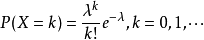1. 在任意单位时间长度内，到达率是稳定的。
2. 未来的实验结果与过去的实验结果无关。
3. 在极小的一段时间内，有1次到达的概率非常小，没有到达的概率非常大。

## 泊松分布与指数分布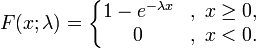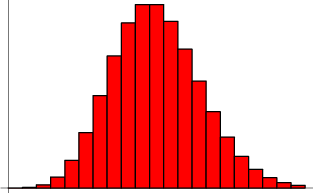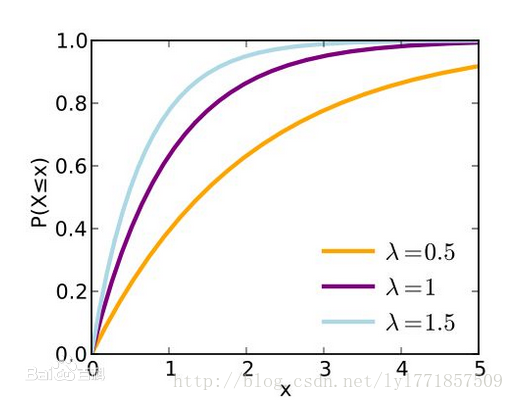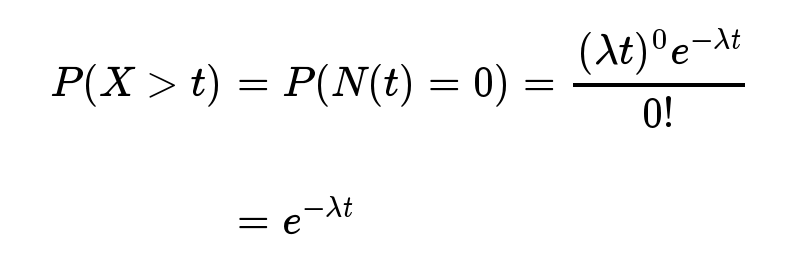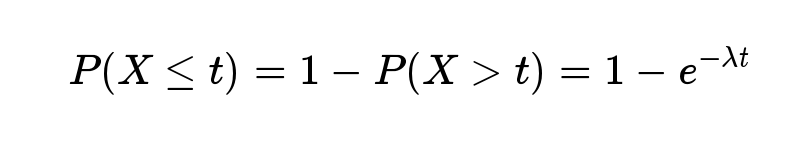#### 与伽马分布的关系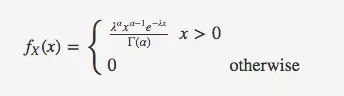## 二项分布和泊松分布

n很大，p很小时泊松分布可以用来近似二项分布，此时 λ=np

2018-08-26 17:48:46 cherrylvlei 阅读数 8138

# 泊松分布$P={C}_{n}^{k}×{p}^{k}×\left(1-p{\right)}^{n-k}$$P=\underset{n\to \infty }{lim}{C}_{n}^{k}×{p}^{k}×\left(1-p{\right)}^{n-k}$

$\begin{array}{rl}P=& \underset{n\to \infty }{lim}{C}_{n}^{k}×\left(\frac{\mu }{n}{\right)}^{k}×\left(1-\frac{\mu }{n}{\right)}^{n-k}\\ P=& \underset{n\to \infty }{lim}\frac{n\left(n-1\right)\left(n-2\right)...\left(n-k+1\right)}{k!}\frac{{\mu }^{k}}{{n}^{k}}\left(1-\frac{\mu }{n}{\right)}^{n-k}\\ P=& \underset{n\to \infty }{lim}\frac{{\mu }^{k}}{k!}\frac{n\left(n-1\right)...\left(n-k+1\right)}{{n}^{k}}\left(1-\frac{\mu }{n}{\right)}^{-k}\left(1-\frac{\mu }{n}{\right)}^{n}\end{array}$

$\begin{array}{rl}\underset{n\to \infty }{lim}& \frac{n\left(n-1\right)...\left(n-k+1\right)}{{n}^{k}}\left(1-\frac{\mu }{n}{\right)}^{-k}=1\\ \underset{n\to \infty }{lim}& \left(1-\frac{\mu }{n}{\right)}^{n}={e}^{-\mu }\end{array}$

$\begin{array}{rl}{P}_{k}=& \underset{n\to \infty }{lim}{C}_{n}^{k}×{p}^{k}×\left(1-p{\right)}^{n-k}\\ \text{(6)}& =& \frac{{\mu }^{k}}{k!}{e}^{-\mu }\end{array}$$\sum _{k=1}^{n}{P}_{k}=\sum _{k=1}^{n}\frac{{100}^{k}}{k!}{e}^{-100}>1-10\mathrm{%}=0.9$

# 结语2015-12-15 19:19:04 qq_18343569 阅读数 8221clc,clear,close all
warning off
feature jit off
Z1 = imnoise_poission(im,size(im,1),size(im,2),0.3);
Z1 = im2uint8(Z1);   % 类型转换
figure('color',[1,1,1]),
im(:,:,1) = im(:,:,1) + Z1;  % R
im(:,:,2) = im(:,:,2) + Z1;  % G
im(:,:,3) = im(:,:,3) + Z1;  % B
subplot(121); imshow(im);title('加泊松分布噪声图像')
subplot(122); imhist(Z1); title('加泊松分布噪声图像直方图')



function R = imnoise_poission(im,M, N, lamda)
% input:
%       泊松poission分布，噪声的类型；
%       M,N：输出噪声图像矩阵的大小
%       a,b：各种噪声的分布参数
% output:
%       R: 输出的噪声图像矩阵，数据类型为double型
% 设定默认值
if nargin < 4
lamda = 0.5;
end
% 产生poission分布噪声
for i = 1:M
for j=1:N
b=1;
c = double( floor(im(i,j)/30)+1 );
for k =1:c
b=b*k;
end
R(i,j) = exp(-lamda).*lamda.^(c)./b;
end
end
end